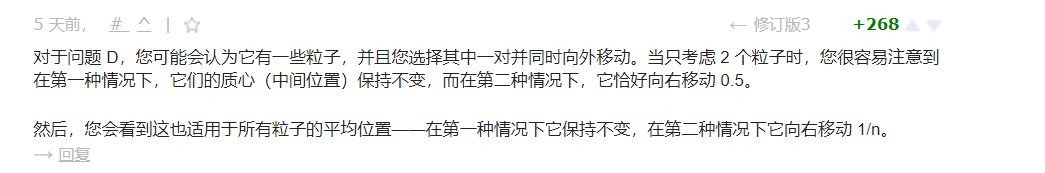div2第一次A了三个题，加了五十多分，我离小蓝又近了一步~

A题：大意是给出一段01串，每次我们可以使得前两个数字中的第二个数字替换成前两个数的一个，然后删除第一个数字。

code：

```#include<bits/stdc++.h>
using namespace std;
#pragma GCC optimize(2)

int main()
{
ios_base::sync_with_stdio(0); cin.tie(0); cout.tie(0);
int t;
cin>>t;
string a,b;
vector<int>ve;
while(t--)
{
a.clear();
b.clear();
ve.clear();
int n,m;
cin>>n>>m;
cin>>a>>b;
reverse(a.begin(),a.end());
reverse(b.begin(),b.end());
int i=0;
for(;i<b.size();i++)
{
if(b[i]!=a[i])
break;
}
if(i==b.size())
{
printf("YES\n");
}else if(i==b.size()-1)
{
char t = b[m-1];
int j=m-1;
for(;j<a.size();j++)
{
if(a[j]==t)
break;
}
if(j<a.size())
printf("YES\n");
else
printf("NO\n");
}else
printf("NO\n");
}

}

```

B题：大意是有n堆食物，每堆食物存在一个亲和力，刚开始小明亲和力可以为任意一个数，每次吃食物要保证亲和力与食物的亲和力的差绝对值<=x。询问，在吃完所有食物后，亲和力最少的改变次数。思路：对于一个区间，只要最大值-最小值小于等于2*x，那么我们可以将亲和力设置为中间数来吃掉区间内的所有食物。

code:

```#include<bits/stdc++.h>
using namespace std;
#pragma GCC optimize(2)
#define ll long long
vector<ll>ve;
int main()
{
ios_base::sync_with_stdio(0); cin.tie(0); cout.tie(0);
int t;
cin>>t;
while(t--)
{
ve.clear();
ll n,x,xx,sum=0;
cin>>n>>x;
for(int i=1;i<=n;i++)
{
cin>>xx;
ve.push_back(xx);
}
for(int i=0;i<ve.size();)
{
int tt = i;
ll maxt = max(ve[tt],ve[i]),mint = min(ve[tt],ve[i]);
do{
tt++;
maxt = max(maxt,ve[tt]);
mint = min(mint,ve[tt]);
}while(maxt - mint<=x*2&&tt<ve.size());
if(tt!=ve.size())
sum++;
i = tt;
}
cout<<sum<<endl;
}
}```

C题：大意是1-n的房屋围成了一个圈，每天我们可以选择保护一个房子使其永久免疫病毒，同时每天感染病毒房子会向两边的房子扩散，使其被感染，询问，我们最少可以使得多少房屋被感染。思路：既然形成了一个圈，那么我们可以求各个圈内房屋的数量，对于一个区间内房屋的数量qn，我们第一次可以使得qn-1的房屋免受感染，第一天我们选择一个边界屋，然后病毒扩散另一个边界一个屋子，我们再选择另一边界，即可保护qn-1个房屋。对于其他区间，我们需要计算保护之前区间所用时间被感染的房子，然后再保护区间内房屋。每次我们保护一个区间需要两天时间，两天时间一个区间会被感染新的4个房屋。我们可以累加这个数量，或者与循环变量相乘。我们减去这个数再减1就是能保护的房屋。根据贪心的思想，我们应该优先保护尽可能多的房屋，使得被保护的房屋数量值最大。将所有边界保护起来，病毒无法扩散就形成不了新的被感染的房屋。

code：

```#include<bits/stdc++.h>
using namespace std;
#pragma GCC optimize(2)
vector<int>ve;
vector<int>s;
bool cmp(int a,int b)
{
return a>b;
}
int main()
{
ios_base::sync_with_stdio(0); cin.tie(0); cout.tie(0);
int t;
cin>>t;
while(t--)
{
ve.clear();
s.clear();
int n,m,x;
cin>>n>>m;
for(int i=1;i<=m;i++)
{
cin>>x;
ve.push_back(x);
}
sort(ve.begin(),ve.end());
int ans = 0;
for(int i=0;i<ve.size()-1;i++)
s.push_back(ve[i+1]-ve[i]-1);
int tsize = ve.size()-1;
int tf = (n-ve[tsize])+ve-1;
s.push_back(tf);

sort(s.begin(),s.end(),cmp);

/*	for(int i=0;i<s.size();i++)
cout<<s[i]<<' ';
cout<<endl;*/

int sum = 0,t =1;
for(int i=0;i<s.size();i++)
{
if(s[i]-t<=0)
{
if(s[i]-t==0)
sum++;
break;
}
sum+=s[i]-t;
t+=4;
}

cout<<n-sum<<endl;
}
}

```

D题：大意是给出n个数组。对于普通数组，可以选择i ,j 使得a[i]和a[j]减少1，a[i-1]和a[i+1]增加1. 对于特殊数组，可以选择i ,j 使得a[i]和a[j]减少1，a[i-1]和a[i+2]增加1. 求出哪个是特殊数组。特殊数组经过了多少次变化。我们考虑另一种想法，以前缀和观念来看，特殊数组由于元素大小右移动，所以利用前缀和的和加起来肯定要比普通数组要小，因为有些靠后的元素加的次数少。

//每次执行操作2，受影响的元素它们的局部质心右移了0.5个
//对于每次局部质心右移了0.5个单位，一定会导致在操作2的局部质心位置的前缀和比操作1这个位置的前缀和少1

```#include<bits/stdc++.h>
using namespace std;
#pragma GCC optimize(2)
#define ll long long
const int nn = 1e5+5;
ll num[nn];
ll t,n,m,maxt,x;
int main()
{
ios_base::sync_with_stdio(0); cin.tie(0); cout.tie(0);
int t;
cin>>t;
while(t--)
{
memset(num,0,sizeof num);
cin>>n>>m;
for(int i=1;i<=n;i++)
for(int j=1;j<=m;j++)
{
cin>>x;
num[i]+=j*x;
}
/*for(int i=1;i<=n;i++)
cout<<num[i]<<' ';
cout<<endl;*/
maxt = max_element(num+1,num+n+1) - num;
cout<<maxt<<' '<<num[maxt]-num[(maxt==1?2:1)]<<endl;
}
}

```

```#include<bits/stdc++.h>
using namespace std;
#pragma GCC optimize(2)
#define ll long long
const int nn = 1e5+5;
ll s[nn];
ll n,m,x;
int main()
{
ios_base::sync_with_stdio(0); cin.tie(0); cout.tie(0);
int t;
cin>>t;
while(t--)
{
memset(s,0,sizeof s);
cin>>n>>m;
for(int i=1;i<=n;i++)
{
ll sum = 0,pos = 0;
for(int j=1;j<=m;j++)
{
cin>>x;
pos+=x;
sum+=pos;
}
s[i] = sum;
}
int mint = min_element(s+1,s+n+1) - s;
cout<<mint<<" "<<s[(mint==1?2:1)]-s[mint]<<endl;
}
}

```